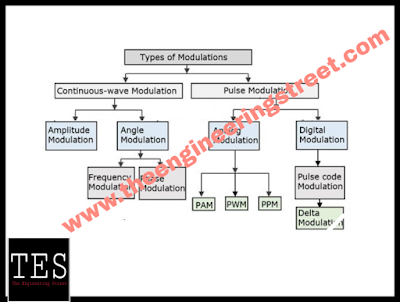# Classification Of Pulse Code Modulation Difference Between Analog And Digital Pulse Code Modulation!

## Here In Article Contain Classification Of Pulse Code Modulation And Difference Between Analog And Digital Pulse Code Modulation Pulse Transmitter And Pulse Receiver

#### Pulse Code Modulation:

First of all we learn about pulse modulation and its types , After that shows PCM(Pulse Code Modulation ).

In pulse modulation, the carrier is in the form of train of periodic rectangular pulses.

Pulse modulation can be either analog or digital.

In analog pulse modulation, the amplitude ,width or position of the rectangular pulses is changed in accordance with the modulating signal.

This will result in PAM(pulse amplitude modulation), PWM (pulse width modulation),or PPM(pulse position modulation).

The pulse modulation is also can be digital . The known examples of digital pulse modulation are pulse code modulation(PCM),delta modulation(DM),adaptive delta modulation(ADM),etc.

The classification of the pulse modulation system is as follow:Types Of Pulse Code Modulation

#### 1. Pulse Amplitude Modulation:

The amplitude of a constant width,constant position,rectangular carrier is varied with the instantaneous magnitude of modulating signal as shown in below figure.

#### 2. Pulse Width Modulation:

The width of carrier pulses is made to vary in proportion with the instantaneous magnitude of the modulating signal as shown in below figure.

PWM is also known as pulse duration modulation(PDM) or pulse length modulation(PLM).

#### 3. Pulse Position Modulation:

In PPM the amplitude and width of the pulses is constant but the position of each pulse is varied in accordance with the amplitudes of the sampled values of the modulating signal.

The position of the pulses is changed with respect to the position of reference pulses.

The PPM can be derived from the PWM  pulse as shown in figure.

Note that with increase in the modulating voltage the PPM pulses shift further with respect to reference.

#### 4. Pulse Code Modulation:

The analog message signal is sampled and covered to a fixed length, serial binary number as shown in figure.

In other words a binary code is transmitted. Hence the name pulse code modulation.

The PAM,PWM  and PPM are called analog pulse communication systems whereas PCM ,delta modulation(DM) are the examples of digital pulse communication systems.Waveform Of Pulse Code Modulation

### What are the difference between analog and digital pulse communication??

For analog as well as digital pulse communication systems, the transmitted signal is a discrete time signal.

In analog pulse communication the information is transmitted in the form of change in amplitude,width and position of the rectangular carrier pulse.

So the transmitted pulsed signal is still analog signal.

In digital pulse communication the information is transmitted in the form of codes.

code word are formed by grouping the digital pulses.

Note that for digital pulse communication we do not change amplitude , frequency or phase of the transmitted signal.

Thus the transmitted signal in digital pulse communication is a digital signal.

### Pulse Code Modulation(PCM):

Basis of PCM shows as above classification......

The information is transmitted in the form of "code words".

A PCM system consists of a PCM encoder(transmitter) and a PCM decoder(receiver).

### PCM Transmitter (Encoder):

Block diagram of the PCM transmitter is shown in below figure.

• Operation of PCM transmitter:

The analog signal x(t) is passed through a bandlimiting low pass filter, which has a cut-off frequency fc  = W Hz.

The bandlimited analog signal is then applied to a sample and hold circuit where it is sampled at high  sampling rate.output of sample and hold block is a flat topped PAM signal.

These samples are then subject to the operation "Quantization" in the "Quantizer".

Quantization is used to reduce the effect of noise.

The combined effect of sampling and quantization produces the quantized PAM at the quantizer output.

The quantized PAM pulses are applied to an encoder which is basically an A to D converter.

Each quantized level is converted into an N bit digital word by the A to D converter.

The encoder output is converter into a stream of pulses by the parallel to the serial converter block.Thus at the PCM transmitter output we get a train of digital pulses.

A pulse generator produces a train of rectangular pulses with each pulse of duration T seconds.Basic Block Diagram Of PCM Receiver

Above figure shows the block diagram of a PCM receiver.

A PCM signal contaminated with the noise is available at the receiver input.

The regeneration circuit at the receiver will separate the the PCM pulses from noise and will reconstruct the original PCM signal.

The pulse generator has to operate in synchronization with that at the transmitter. thus at the regeneration circuit output we get a "clean" PCM signal.

The reconstruction of PCM signal is possible due to the digital nature of PCM signal.

The reconstructed PCM signal is then passed through a serial to parallel converter.

Output of this block is then applied to a decoder.

The decoder is a D to A converter which performs exactly the opposite operation of the encoder.

The decoder output is the sequence of a quantized multilevel pulses.

The quantized PAM signal is thus obtained, at the output of the decoder.

This quantized PAM signal is passed through a low pass filter to recover the analog signal x(t).

The low pass filter is called as the reconstruction filter and its cut off frequency is equal to the message bandwidth W.

May You Like To Read This More Related Informative Articles On Electronics Engineering :